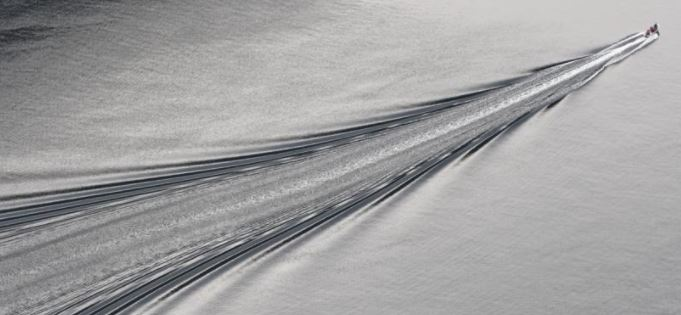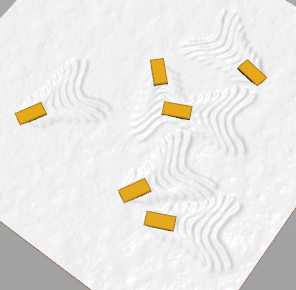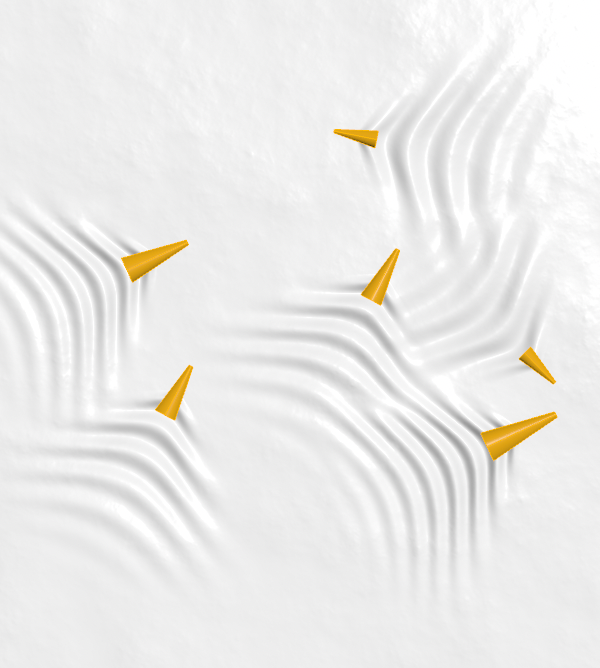## Recommended Posts

Hello.

I want to make a ripple like image.

If you do not use the ripple solver (without simulation) is there any way i can make it?

If you have any good ideas, please share them with me.

I wish I could customize the length, width...etc of the ripple. thank you.##### Share on other sites

Hi @eunchae,

you could copy and transfer displaced grid rows into a height field:boat_waves.hiplc

##### Share on other sites

Alternatively remap and average out directions and distances to points across a height field:```float scale = chf('scale');
float range = chf('range');

float disps[] = {};
int pts[] = nearpoints(1, v@P, range * 4.0);
foreach(int pt_near; pts){
vector pos = point(1, 'P', pt_near);
vector nml = point(1, 'N', pt_near);
float pscale = point(1, 'pscale', pt_near);
vector dir = normalize(pos - v@P);
float angle = fit11(dot(nml, dir), 0.0, 1.0);
float dist = distance(pos, v@P);
float shape = chramp('shape', angle);
float dist_mod = dist * shape * pscale;
float mask = 1.0 - smooth(0.0, range, dist);
float wave = sin(dist_mod * 0.02);
float height = 1.0 - chramp('height', angle);
float disp = height * mask * scale * wave;
append(disps, disp);
}

f@height = avg(disps);```

boat_waves_VEX.hiplc

•2

##### Share on other sites
On 2021. 9. 27. at 6:05 PM, konstantin magnus said:

Alternatively remap and average out directions and distances to points across a height field:```
float scale = chf('scale');
float range = chf('range');

float disps[] = {};
int pts[] = nearpoints(1, v@P, range * 4.0);
foreach(int pt_near; pts){
vector pos = point(1, 'P', pt_near);
vector nml = point(1, 'N', pt_near);
float pscale = point(1, 'pscale', pt_near);
vector dir = normalize(pos - v@P);
float angle = fit11(dot(nml, dir), 0.0, 1.0);
float dist = distance(pos, v@P);
float shape = chramp('shape', angle);
float dist_mod = dist * shape * pscale;
float mask = 1.0 - smooth(0.0, range, dist);
float wave = sin(dist_mod * 0.02);
float height = 1.0 - chramp('height', angle);
float disp = height * mask * scale * wave;
append(disps, disp);
}

f@height = avg(disps);```

Thank you. If I have to ask you one more thing, can you make it like the last pattern in the video? Even if I use ripple sop, such a shape does not come out.

##### Share on other sites

Hi @eunchae,

@ssh has already implemented the Kelvin Wake pattern in Houdini:

•1
•1

##### Share on other sites
9 hours ago, konstantin magnus said:

Hi @eunchae,

@ssh has already implemented the Kelvin Wake pattern in Houdini:

Thank you!

## Create an account or sign in to comment

You need to be a member in order to leave a comment

## Create an account

Sign up for a new account in our community. It's easy!

Register a new account